LDP LLC - MaxMax.com

Desert Study

You can jump to the Remote Sensing Section of our online store by clicking here.

Goal:  Identify and quantify vegetation growing in the desert and estimate biomass.

Equipment Used:  Canon550NDVI MK II vegetation stress camera

Software Used: ImageJ with MaxMax Enhanced NDVI (ENDVI) macro

Normal visual picture of area.

Notice that you can see some brownish plants which are likely quite stressed as well, but it is pretty hard to see the difference between the plants and the dirt..  For this study, we want to be able to pick out vegetation.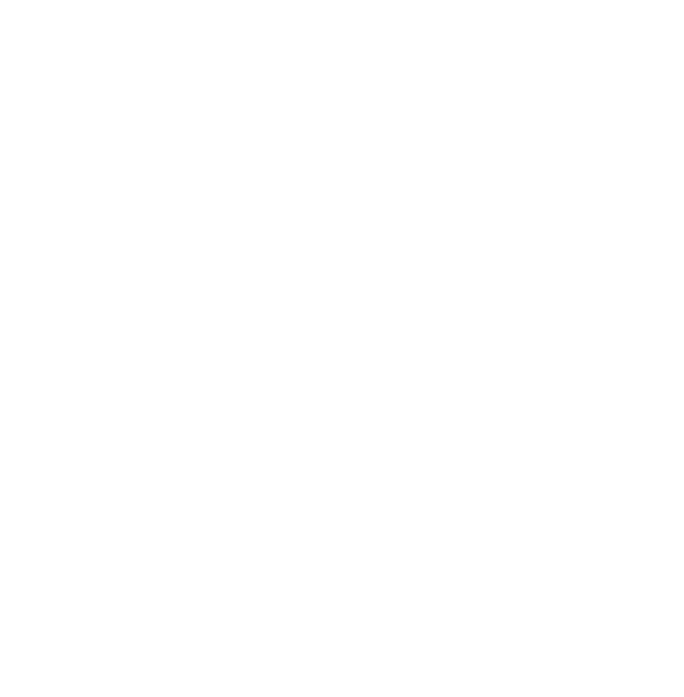Unprocessed picture from the Canon550NDVI MK II camera.

Notice that the plants now have a reddish tint.  This is because the plants reflect the near IR which is picked up by the camera in the red channel.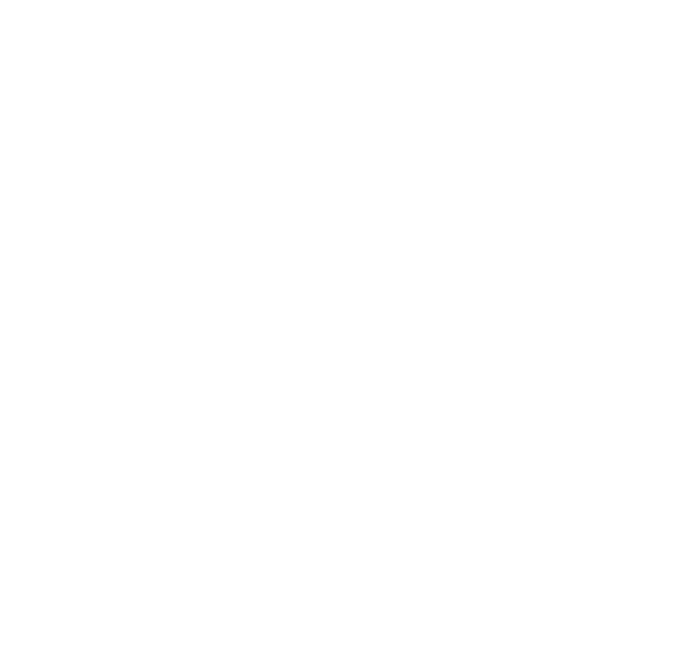Canon550NDVI MK II image processed with ImageJ using macro aNDVI6_MKII

The macro uses our Enhanced NDVI (ENDVI) calculation that uses all 3 bands.  This ENDVI uses

ENDVI = ((Red + Green ) -2*Blue) / ((Red + Green) + 2*Blue))

The logic behind this NDVI calculation is that plants will reflect both green and the near IR, which is in the red channel.  Plants absorb blue light so the blue channel is used as the visible channel and is multiplied by 2 to compensate for the 2 plant reflective channels.

The macro then further processes the data by rescaling the NDVI values to a user set maximum and minimum.  The reason for this is that if the maximum plants for this sort of picture have an NDVI of 0.50 while the rocks have a value of -0.15, most of the NDVI value in the picture will be between -0.15 and +0.50.  By rescaling the ENDVI values so that -0.15 = -1.0 and +0.50 = +1.0, then we can get a better understanding about what is in the picture.

Next the macro takes the rescaled ENDVI values and maps each pixel in the picture so that the closer to +1.0, the more green the pixel and the closer to -1.0, the more blue the pixel becomes.  A new image is created where each pixel is a color representation of the rescaled NDVI values where green is mapped to the vegetation and blue is matched to stuff that isn't.

Notice that now we can easily pick out the areas with plants.  We can also see that the rocks are generally blue while the dirt is generally black.

Default values for rescaling the NDVI values was minimum value of -0.15 and maximum value of +0.50.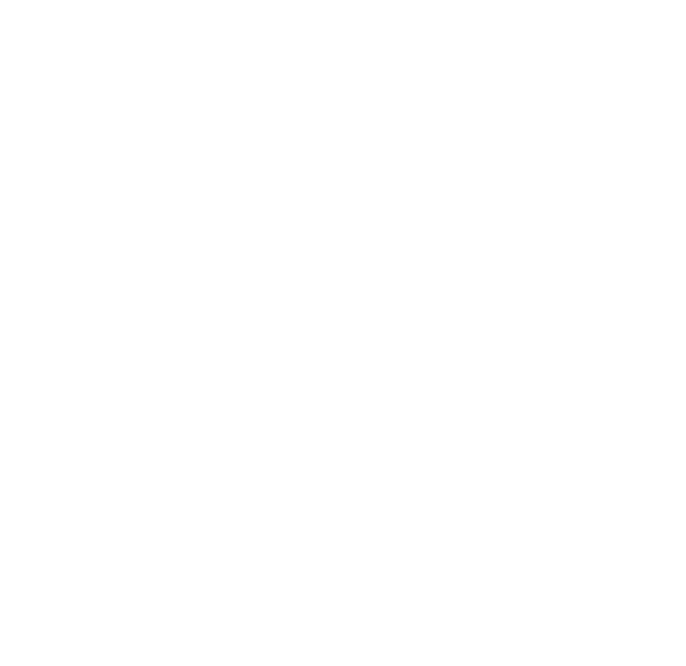The macro measures each pixel and keeps a count of every 0.10 between -1.00 and +1.00 to summarize the picture.  You could use this to calculate the total vegetation in the picture, the stress of the vegetation, the amount of rocks and the amount of dirt.a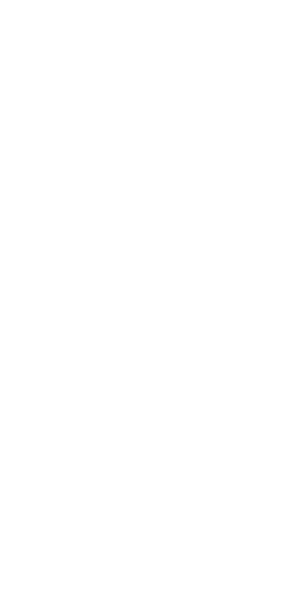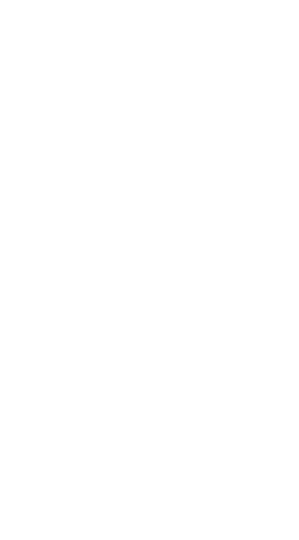Lastly, here is a copy of the ImageJ macro that we used:

// ENDVI 6 Macro
// Written by Dan Llewellyn
// www.MaxMax.com
// For NDVI MK II Cameras
// Copywrite 2011
// This macro converts an RGB image to a Green/Blue NDVI image.
// NDVI values > 0 are colored green with user set max value
// NDVI values < 0 are colored blue with user set min value
// This macro automatically rescales the image so that max value is 100% green and min value is 100% blue
// Green shows healthy plants.
// Blue represents negative NDVI values which are often things like dirt and rocks.

NDVI_Plant_Max = 0.50;        // Set the highest value for a NDVI plant in picture
NDVI_Not_A_Plant = -0.15;     // Set to highest value for not a plant - max negative -.15
ScaledNDVI = 0;               // NDVI scaled to max value
///////////////////////////////////////////
//  Statistics Counters
//////////////////////////////////////////
//  Total counters for scaled NDVI values
TotalScaledNDVI_9_10  = 0;     // Total scaled NDVI +0.90 to +1.00
TotalScaledNDVI_8_9   = 0      // Total scaled NDVI +0.80 to +0.90
TotalScaledNDVI_7_8   = 0;     // Total scaled NDVI +0.70 to +0.80
TotalScaledNDVI_6_7   = 0;     // Total scaled NDVI +0.60 to +0.70
TotalScaledNDVI_5_6   = 0;     // Total scaled NDVI +0.50 to +0.60
TotalScaledNDVI_4_5   = 0;     // Total scaled NDVI +0.40 to +0.50
TotalScaledNDVI_3_4   = 0;     // Total scaled NDVI +0.30 to +0.40
TotalScaledNDVI_2_3   = 0;     // Total scaled NDVI +0.20 to +0.30
TotalScaledNDVI_1_2   = 0;     // Total scaled NDVI +0.10 to +0.20
TotalScaledNDVI_0_1   = 0;     // Total scaled NDVI +0.00 to +0.10
TotalScaledNDVI_0_n1  = 0;     // Total scaled NDVI +0.00 to -0.10
TotalScaledNDVI_n1_n2 = 0;     // Total scaled NDVI -0.10 to -0.20
TotalScaledNDVI_n2_n3 = 0;     // Total scaled NDVI -0.20 to -0.30
TotalScaledNDVI_n3_n4 = 0;     // Total scaled NDVI -0.30 to -0.40
TotalScaledNDVI_n4_n5 = 0;     // Total scaled NDVI -0.40 to -0.50
TotalScaledNDVI_n5_n6 = 0;     // Total scaled NDVI -0.50 to -0.60
TotalScaledNDVI_n6_n7 = 0;     // Total scaled NDVI -0.60 to -0.70
TotalScaledNDVI_n7_n8 = 0;     // Total scaled NDVI -0.70 to -0.80
TotalScaledNDVI_n8_n9 = 0;     // Total scaled NDVI -0.80 to -0.90
TotalScaledNDVI_n9_n10 = 0;    // Total scaled NDVI -0.90 to -1.00
TotalScaledPixelPercent = 0;   // This will be used later to calculate percent of all pixels for each Total Scaled Count
MaxNDVI = 0;                    // Maximum NDVI
MinNDVI = 0;                    // Minimum NDVI
MaxScaledNDVI = 0;              // Maximum Scaled NDVI
MinScaledNDVI = 0;              // Minimum Scaled NDVI
TotalPixels = 0;                // Total Pixels
/////////////////////////////////////////////
requires("1.29m");          // Check ImageJ version
w = getWidth();             // Get picture width
h = getHeight();            // Get picture height
start = getTime();                                                   // Get current time for progress bar

for (y=0; y<h; y++)                                               // Setup nested loops to go through each x,y value in image
{
for (x=0; x<w; x++)
{
oldpixel = getPixel(x,y);                                   // Get a pixel value
red =   (oldpixel & 0xff0000)>>16;                          // Extract out RGB values from packed integer with pixel value
green = (oldpixel & 0x00ff00)>>8;
blue =  (oldpixel & 0x0000ff);
NDVI = ((red+green)-(2*blue))/((red+green)+(2*blue));       // Calculate the NDVI.  Will be a value between -1.0 and + 1.0
if (NDVI < 0)                                               // not a plant
{
ScaledNDVI = NDVI/(-1*NDVI_Not_A_Plant);                 // Calc NDVI as a % of max value.
if (ScaledNDVI < -1) ScaledNDVI = -1;                    // Make sure not going over limit for max.  Max NDVI value is +1.0
blue= floor(ScaledNDVI*-255);                            // Set red value and rescale to max 255 bit depth.
red = 0;
green = 0;

}
else                                                        // Possible plant
{
ScaledNDVI = NDVI/NDVI_Plant_Max;                        // Calc NDVI as a % of max value.
if (ScaledNDVI > 1) ScaledNDVI = 1;                      // Make sure not going over limit for max.  Max NDVI value is +1.0
green = floor(ScaledNDVI*255);                           // set green value and rescale to max 255 bit depth.
blue = 0;
red = 0;
}
newpixel = ((red & 0xff)<<16)+((green & 0xff)<<8) + (blue & 0xff);  // Pack new pixel integer value with new RGB data
putPixel(x, y, newpixel);                                   // write pixel to the screen

//  Statistics Collection
if (NDVI > MaxNDVI) MaxNDVI = NDVI;                          // Test for Max NDVI
if (NDVI < MinNDVI) MinNDVI = NDVI;                          // Test for Min NDVI
if (NDVI > MaxScaledNDVI) MaxScaledNDVI = NDVI;              // Test for Max Scaled NDVI
if (NDVI < MinScaledNDVI) MinScaledNDVI = NDVI;              // Test for Min Scaled NDVI

if (ScaledNDVI > 0.90)                                       //  Keep track of each pixels value by binning it into correct counter
TotalScaledNDVI_9_10 ++;
else if (ScaledNDVI > 0.80 && ScaledNDVI < 0.90)
TotalScaledNDVI_8_9 ++;
else if (ScaledNDVI > 0.70 && ScaledNDVI < 0.80)
TotalScaledNDVI_7_8 ++;
else if (ScaledNDVI > 0.60 && ScaledNDVI < 0.70)
TotalScaledNDVI_6_7 ++;
else if (ScaledNDVI > 0.50 && ScaledNDVI < 0.60)
TotalScaledNDVI_5_6 ++;
else if (ScaledNDVI > 0.40 && ScaledNDVI < 0.50)
TotalScaledNDVI_4_5 ++;
else if (ScaledNDVI > 0.30 && ScaledNDVI < 0.40)
TotalScaledNDVI_3_4 ++;
else if (ScaledNDVI > 0.20 && ScaledNDVI < 0.30)
TotalScaledNDVI_2_3 ++;
else if (ScaledNDVI > 0.10 && ScaledNDVI < 0.20)
TotalScaledNDVI_1_2 ++;
else if (ScaledNDVI > 0.00 && ScaledNDVI < 0.10)
TotalScaledNDVI_0_1 ++;
else if (ScaledNDVI > -0.10 && ScaledNDVI < 0)
TotalScaledNDVI_0_n1 ++;
else if (ScaledNDVI > -0.20 && ScaledNDVI < -0.10)
TotalScaledNDVI_n1_n2 ++;
else if (ScaledNDVI > -0.30 && ScaledNDVI < -0.20)
TotalScaledNDVI_n2_n3 ++;
else if (ScaledNDVI > -0.40 && ScaledNDVI < -0.30)
TotalScaledNDVI_n3_n4 ++;
else if (ScaledNDVI > -0.50 && ScaledNDVI < -0.40)
TotalScaledNDVI_n4_n5 ++;
else if (ScaledNDVI > -0.60 && ScaledNDVI < -0.50)
TotalScaledNDVI_n5_n6 ++;
else if (ScaledNDVI > -0.70 && ScaledNDVI < -0.60)
TotalScaledNDVI_n6_n7 ++;
else if (ScaledNDVI > -0.80 && ScaledNDVI < -0.70)
TotalScaledNDVI_n7_n8 ++;
else if (ScaledNDVI > -0.90 && ScaledNDVI < -0.80)
TotalScaledNDVI_n8_n9 ++;
else if (ScaledNDVI  < -0.90)
TotalScaledNDVI_n9_n10 ++;

TotalPixels ++;                                         // Count another pixel
}
if (y%10==0) showProgress(y, h-1);                          // show current progress
if (y%10==0) updateDisplay();
}
showStatus(round((w*h)/((getTime()-start)/1000)) + " pixels/sec");
resetMinAndMax();

Dialog.create("NDVI Summary");                                  // Create the pixel count dialog.  Display total in counters.
Dialog.addNumber("Scaled NDVI Pixels 0.90 to 1.00", TotalScaledNDVI_9_10);
Dialog.addNumber("Scaled NDVI Pixels 0.80 to 0.90", TotalScaledNDVI_8_9);
Dialog.addNumber("Scaled NDVI Pixels 0.70 to 0.80", TotalScaledNDVI_7_8);
Dialog.addNumber("Scaled NDVI Pixels 0.60 to 0.70", TotalScaledNDVI_6_7);
Dialog.addNumber("Scaled NDVI Pixels 0.50 to 0.60", TotalScaledNDVI_5_6);
Dialog.addNumber("Scaled NDVI Pixels 0.40 to 0.50", TotalScaledNDVI_4_5);
Dialog.addNumber("Scaled NDVI Pixels 0.30 to 0.40", TotalScaledNDVI_3_4);
Dialog.addNumber("Scaled NDVI Pixels 0.20 to 0.30", TotalScaledNDVI_2_3);
Dialog.addNumber("Scaled NDVI Pixels 0.10 to 0.20", TotalScaledNDVI_1_2);
Dialog.addNumber("Scaled NDVI Pixels 0.00 to 0.10", TotalScaledNDVI_0_1);
Dialog.addNumber("Scaled NDVI Pixels -0.10 to 0.00", TotalScaledNDVI_0_n1);
Dialog.addNumber("Scaled NDVI Pixels -0.20 to -0.10", TotalScaledNDVI_n1_n2);
Dialog.addNumber("Scaled NDVI Pixels -0.30 to -0.20", TotalScaledNDVI_n2_n3);
Dialog.addNumber("Scaled NDVI Pixels -0.40 to -0.30", TotalScaledNDVI_n3_n4);
Dialog.addNumber("Scaled NDVI Pixels -0.50 to -0.40", TotalScaledNDVI_n4_n5);
Dialog.addNumber("Scaled NDVI Pixels -0.60 to -0.50", TotalScaledNDVI_n5_n6);
Dialog.addNumber("Scaled NDVI Pixels -0.70 to -0.60", TotalScaledNDVI_n6_n7);
Dialog.addNumber("Scaled NDVI Pixels -0.80 to -0.70", TotalScaledNDVI_n7_n8);
Dialog.addNumber("Scaled NDVI Pixels -0.90 to -0.80", TotalScaledNDVI_n8_n9);
Dialog.addNumber("Scaled NDVI Pixels -0.10 to -0.90", TotalScaledNDVI_n9_n10);

Dialog.addMessage("\n% Count Summary\n");                      //  Create the % count summary.  Calc binned pixels as % of total and display
TotalScaledPixelPercent = (TotalScaledNDVI_9_10 / TotalPixels)*100;
Dialog.addNumber("Scaled NDVI % 0.90 to 1.00", TotalScaledPixelPercent, 1, 5, "%");
TotalScaledPixelPercent = (TotalScaledNDVI_8_9 / TotalPixels)*100;
Dialog.addNumber("Scaled NDVI % 0.80 to 0.90", TotalScaledPixelPercent, 1, 5, "%");
TotalScaledPixelPercent = (TotalScaledNDVI_7_8 / TotalPixels)*100;
Dialog.addNumber("Scaled NDVI % 0.70 to 0.80", TotalScaledPixelPercent, 1, 5, "%");
TotalScaledPixelPercent = (TotalScaledNDVI_6_7 / TotalPixels)*100;
Dialog.addNumber("Scaled NDVI % 0.60 to 0.70", TotalScaledPixelPercent, 1, 5, "%");
TotalScaledPixelPercent = (TotalScaledNDVI_5_6 / TotalPixels)*100;
Dialog.addNumber("Scaled NDVI % 0.60 to 0.60", TotalScaledPixelPercent, 1, 5, "%");
TotalScaledPixelPercent = (TotalScaledNDVI_4_5 / TotalPixels)*100;
Dialog.addNumber("Scaled NDVI % 0.40 to 0.50", TotalScaledPixelPercent, 1, 5, "%");
TotalScaledPixelPercent = (TotalScaledNDVI_3_4 / TotalPixels)*100;
Dialog.addNumber("Scaled NDVI % 0.30 to 0.40", TotalScaledPixelPercent, 1, 5, "%");
TotalScaledPixelPercent = (TotalScaledNDVI_2_3 / TotalPixels)*100;
Dialog.addNumber("Scaled NDVI % 0.20 to 0.20", TotalScaledPixelPercent, 1, 5, "%");
TotalScaledPixelPercent = (TotalScaledNDVI_1_2 / TotalPixels)*100;
Dialog.addNumber("Scaled NDVI % 0.10 to 0.20", TotalScaledPixelPercent, 1, 5, "%");
TotalScaledPixelPercent = (TotalScaledNDVI_0_1 / TotalPixels)*100;
Dialog.addNumber("Scaled NDVI % 0.00 to 0.10", TotalScaledPixelPercent, 1, 5, "%");
TotalScaledPixelPercent = (TotalScaledNDVI_0_n1 / TotalPixels)*100;
Dialog.addNumber("Scaled NDVI % -0.10 to 0.00", TotalScaledPixelPercent, 1, 5, "%");
TotalScaledPixelPercent = (TotalScaledNDVI_n1_n2 / TotalPixels)*100;
Dialog.addNumber("Scaled NDVI % -0.20 to -0.10", TotalScaledPixelPercent, 1, 5, "%");
TotalScaledPixelPercent = (TotalScaledNDVI_n2_n3 / TotalPixels)*100;
Dialog.addNumber("Scaled NDVI % -0.30 to -0.20", TotalScaledPixelPercent, 1, 5, "%");
TotalScaledPixelPercent = (TotalScaledNDVI_n3_n4 / TotalPixels)*100;
Dialog.addNumber("Scaled NDVI % -0.40 to -0.30", TotalScaledPixelPercent, 1, 5, "%");
TotalScaledPixelPercent = (TotalScaledNDVI_n4_n5 / TotalPixels)*100;
Dialog.addNumber("Scaled NDVI % -0.50 to -0.40", TotalScaledPixelPercent, 1, 5, "%");
TotalScaledPixelPercent = (TotalScaledNDVI_n5_n6 / TotalPixels)*100;
Dialog.addNumber("Scaled NDVI % -0.60 to -0.50", TotalScaledPixelPercent, 1, 5, "%");
TotalScaledPixelPercent = (TotalScaledNDVI_n6_n7 / TotalPixels)*100;
Dialog.addNumber("Scaled NDVI % -0.70 to -0.60", TotalScaledPixelPercent, 1, 5, "%");
TotalScaledPixelPercent = (TotalScaledNDVI_n7_n8 / TotalPixels)*100;
Dialog.addNumber("Scaled NDVI % -0.80 to -0.70", TotalScaledPixelPercent, 1, 5, "%");
TotalScaledPixelPercent = (TotalScaledNDVI_n8_n9 / TotalPixels)*100;
Dialog.addNumber("Scaled NDVI % -0.90 to -0.80", TotalScaledPixelPercent, 1, 5, "%");
TotalScaledPixelPercent = (TotalScaledNDVI_n9_n10 / TotalPixels)*100;
Dialog.addNumber("Scaled NDVI % -1.00 to -0.90", TotalScaledPixelPercent, 1, 5, "%");
Dialog.show();